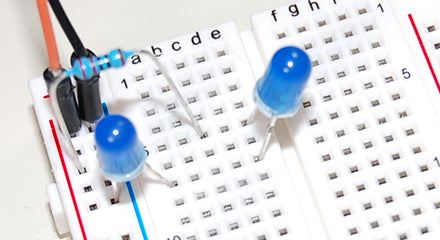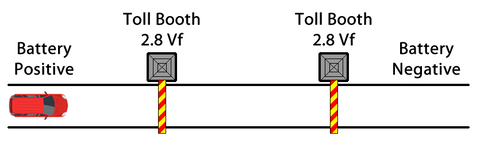# What is Forward Voltage?

This blog post on forward voltage is an excerpt from Lesson A-2 of our Intro to Robotics program. Level A covers building circuits, using a Raspberry Pi, and writing common code commands in Python. It contains 18 lessons including 70+ videos and 45 projects and activities. Sample lessons and a full scope and sequence for Level A can be found here.

When designing and building circuits, one factor you need to consider is forward voltage (Vf). Forward voltage is the minimum amount of voltage that is required to allow an electrical component to turn on.

For example, if you are building a simple circuit containing two LEDs, the amount of forward voltage can vary based on LED color and manufacturer. Generally speaking though, red LEDs are the lowest at 2-volts and white LEDs are the highest at 3-volts. The blue LEDs are around 2.8-volts, so this means each of the LEDs in this circuit will require at least 2.8-volts to turn on.In this example, the LEDs in the circuit are connected in series, so there is only one path from battery positive, through all the components, and back to battery negative. When connected in series the Vf values of the LEDs must be added together:

2.8V + 2.8V = 5.6V

Therefore, to turn on both LEDs would require 5.6-volts. The battery you would typically use for such a circuit is only 3-volts so the LEDs will never receive enough voltage to turn on. Since 3-volts is enough to turn on one LED why won’t at least the first LED turn on?The forward voltage (Vf) in each LED acts almost like a toll booth. For the circuit to be complete you must have enough voltage to pay both tolls. In this case, without at least 5.6V from the battery, neither LED will allow the current to flow. There is no way for the charge to move from its starting point, through both LEDs and onto battery negative (ground). Without current flowing through the circuit, even the first LED will not illuminate.

For this circuit, powering two LEDs in series would require at least 5.6V. However, it’s recommended to also leave a margin of error, so a 6-volt battery would be an appropriate choice for this project. Consequently, keep in mind that anything more than a very simple circuit, operating in series, would require a large amount of voltage to operate. But good news, there is a much more efficient way to connect two LEDs to a power source. It’s called a parallel circuit and you will learn to build one in Lesson A-3 of our Intro to Robotics program.